﻿ 船舶加筋板架结构低噪声优化研究
 舰船科学技术2019, Vol. 41Issue (3): 24-30PDF

1. 中国船舶科学研究中心，江苏 无锡 214082;
2. 深海载人装备国家重点实验室，江苏 无锡 214082

Research on low noise optimization of ship grillages
LIN Chang-gang1,2, SUN Jian-gang1,2, YU Yue1,2, ZHOU Ai-guo1
1. China Ship Scientific Research Center, Wuxi 214082, China;
2. State Key Laboratory of Deep-sea Manned Vehicles, Wuxi 214082, China
Abstract: The acoustic radiation transfer function on initial design plan of pillars in a SWATH is calculated by THAFTS-Acoustic software, and corresponding mode shapes and frequencies whose contribution is largest to the acoustic radiation peaks are separated out. Taking the total level of the vibration response in a frequency interval which contains the mode shapes as objective function, the structure parameters of the pillar grillages are optimized by the ISIGHT and ANSYS software. What's more, the results of optimization plan are validated. Based on the optimization design, several layout plans on damping material are compared, and the low noise optimization design on the SWATH is provided.
Key words: THAFTS-Acoustic     SWATH     acoustic radiation     optimization     damping material
0 引　言

SWATH船体是由连接桥结构连接左右2个片体组成。每一片体包括上船体、支柱体和下潜体，其典型的横剖面如图1所示。由于SWATH船自身的结构特点，在其迎浪或偏迎浪时，海面浅层波浪起伏拍击只对片状支柱起作用，不会造成整个船的排水量明显上下变化，因此，本文以1艘SWATH船支柱体板架结构为研究对象，对结构进行了低噪声的优化设计。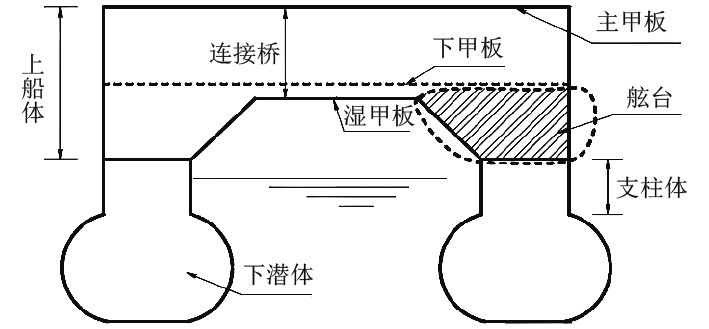图 1 SWATH船典型横剖面示意图 Fig. 1 Typical cross section of a SWATH

1 水中声辐射频域分析方法

 $\vec u = \sum\limits_{r = 1}^m {{{\vec u}_r}{d_r}} = \sum\limits_{r = 1}^m {\{ {u_r},{v_r},{w_r}\} {d_r}} {\text{。}}$ (1)

 $\varPhi (x,y,z,t) = U\bar \varPhi (x,y,z) + \sum\limits_{r = 1}^m {{\phi _r}(x,y,z,t)} {\text{，}}$ (2)

 $\vec W = U\nabla (\bar \varPhi - x){\text{，}}$ (3)

 $\frac{{\partial {\phi _r}}}{{\partial n}} = \vec n \cdot \left[ {{{\dot {\vec u}}_r} + \frac{1}{2}\left( {\nabla \times {{\vec u}_r}} \right) \times \vec W - \left( {{{\vec u}_r} \cdot \nabla } \right)\vec W + \vec W \cdot {\varepsilon _r}} \right]{\text{。}}$ (4)

 ${\left( {i\omega - U\frac{\partial }{{\partial x}}} \right)^2}\phi = 0{\text{，}}$ (5)

 $\phi = 0{\text{。}}$ (6)

 $G(P,Q; - U) = \frac{1}{{4{\text{π}} {r_1}}}{e^{ - ik{r_1}}} - \frac{1}{{4{\text{π}} {r_2}}}{e^{ - ik{r_2}}}{\text{。}}$ (7)

${r_2} = \sqrt {{{(x - \xi )}^2} + {{(y - \eta )}^2} + {{(z + \zeta )}^2}}$ ；　　　 $k = \omega /{c_0}$ 为流体中声波波数， $(x,y,z)$ 为场点 $P$ 的坐标； $(\xi ,\eta ,\varsigma )$ 为源点 $Q$ 的坐标。

 $\begin{split} \phi (P) =\displaystyle\iint_{{\bar{S}}}{\sigma (Q)G(P,Q,-U){\rm d}{{S}_{Q}}} + \displaystyle\frac{1}{g}{U^2}\times \\ \displaystyle\oint_C {{n_1}(Q)\sigma (Q)G(P,Q; - U){\rm d}l} {\text{，}} \end{split}$ (8)

 $[{ a} + { A}] \ddot { d} + [{ b} + { B}] \dot { d} + [{ c} + { C}] { d} = \{ \Xi \} {\text{。}}$ (9)

 $\begin{split} &\left. {\begin{array}{*{20}{c}} {{A_{rk}}}\\ {{B_{rk}}} \end{array}} \right\} = \left. {\begin{array}{*{20}{c}} {\displaystyle\frac{1}{{{\omega ^2}}}{\mathop{\rm Re}\nolimits} }\\ { - \displaystyle\frac{1}{\omega }{\mathop{\rm Im}\nolimits} } \end{array}} \right\}\left[ \rho \displaystyle\iint_{{\bar{S}}}{\vec{n}\cdot {{{\vec{u}}}_{r}}\left( i\omega +\vec{W}\cdot \nabla \right){{\phi }_{k}}{\rm d}S} \right]{\text{，}}\!\!\!\!\!\!\! \\ &{C_{rk}} =-\rho \displaystyle\iint_{{\bar{S}}}{\vec{n}\cdot {{{\vec{u}}}_{r}}\left[ g{{w}_{k}}+\displaystyle\frac{1}{2}\left( {{{\vec{u}}}_{k}}\cdot \nabla \right){{W}^{2}} \right]}{\rm d}S{\text{。}} \end{split}$ (10)

 $p(x,y,z,t) = - \rho \left[ {\frac{\partial }{{\partial t}} + \vec W \cdot \nabla } \right]\phi {\text{。}}$ (11)

 $p(x,y,z,\omega ) = - \rho \left( {i\omega - U\frac{\partial }{{\partial x}}} \right)\phi {\text{，}}$ (12)

 $P(\omega ) = \frac{1}{2}{\mathop{\rm Re}\nolimits} \left\{ {\sum\limits_{k = 1}^m {\sum\limits_{r = 1}^m {i\omega {q_r}{B_{rk}}{{(i\omega {q_k})}^ * }} } } \right\}{\text{，}}$ (13)

2 支柱体初始设计方案声辐射特性分析

SWATH船的支柱体结构的初始设计方案如图2所示，选取船长方向上2个强框架之间的内支柱体板架结构为例，该板架结构由支柱体外板、3根横向球扁钢和2根纵向T型材组成，该长方形板架结构的外壳板厚为13 mm，3根横向加强筋为HP180×8号球扁钢，T型材为 $\displaystyle\frac{{12 \times 150}}{{10 \times 500}}$ mm，密度为7 800 kg/m3，经过计算，板架的总重量为1 334 kg。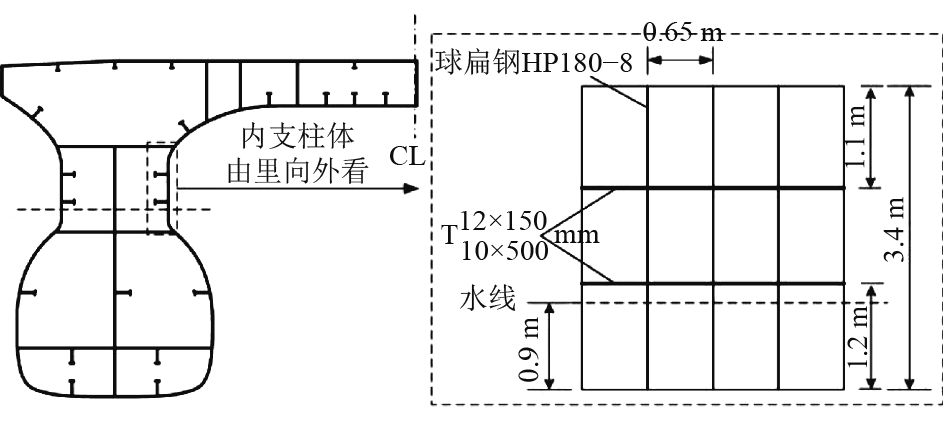图 2 内支柱体初始设计方案 Fig. 2 Initial design plan of the inner pillar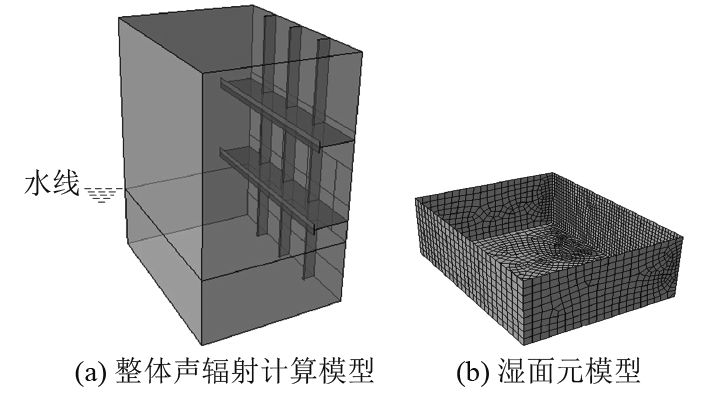图 3 支柱体结构声辐射计算模型 Fig. 3 Acoustic radiation calculation model of pillars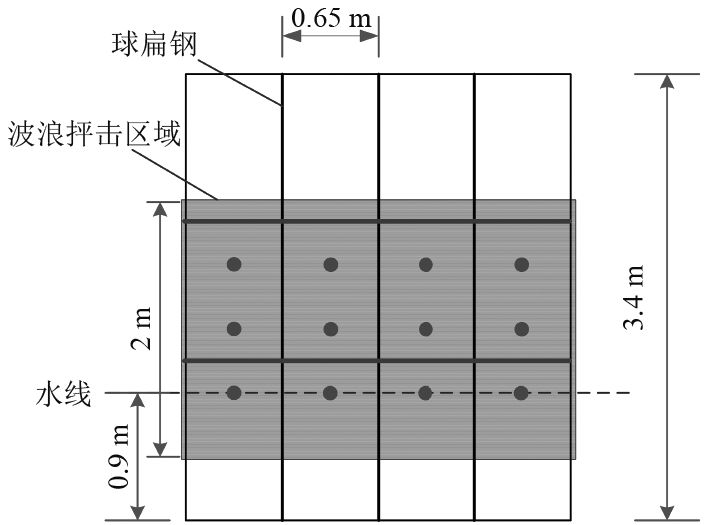图 4 支柱体结构激励点位置 Fig. 4 Positions of exciting force points in pillars
 ${L_s}(\omega ) = 10{\log _{10}}\left( {\frac{{P(\omega )/(4{\text{π}} )}}{{0.65 \times {{10}^{ - 18}}}}} \right){\text{。}}$ (14)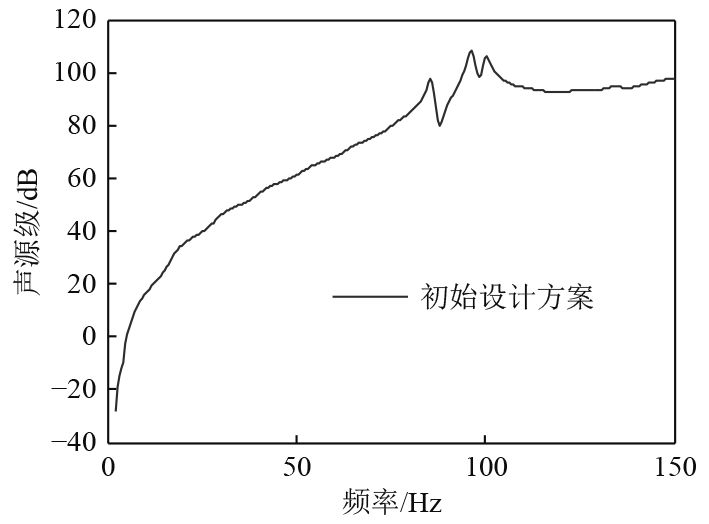图 5 内支柱体初始设计方案声源级结果 Fig. 5 Source level of initial design plan of the inner pillar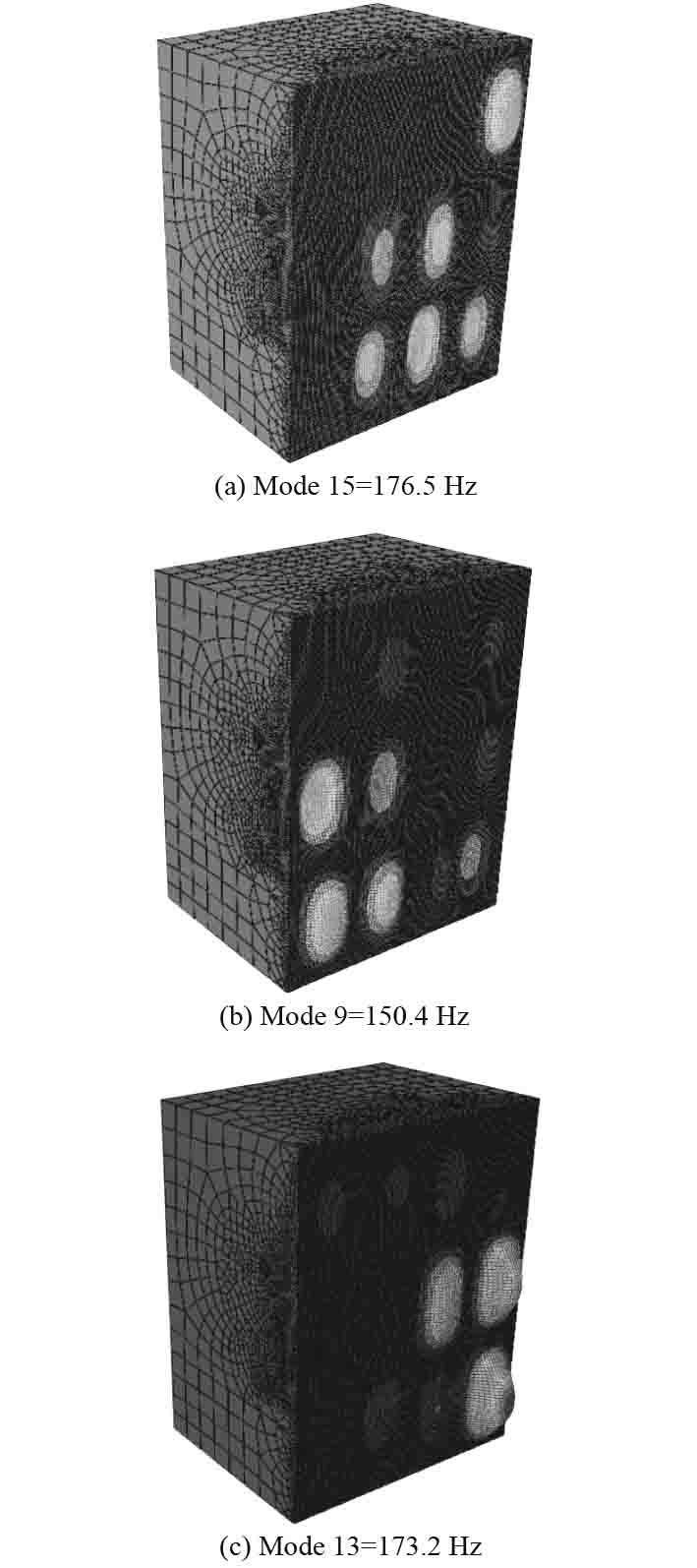图 6 对声辐射峰值贡献最大的干模态振型 Fig. 6 Dry mode shapes with the largest contribution to acoustic radiation peaks
3 支柱体结构声辐射性能优化 3.1 纵向T型材为2根的优化方案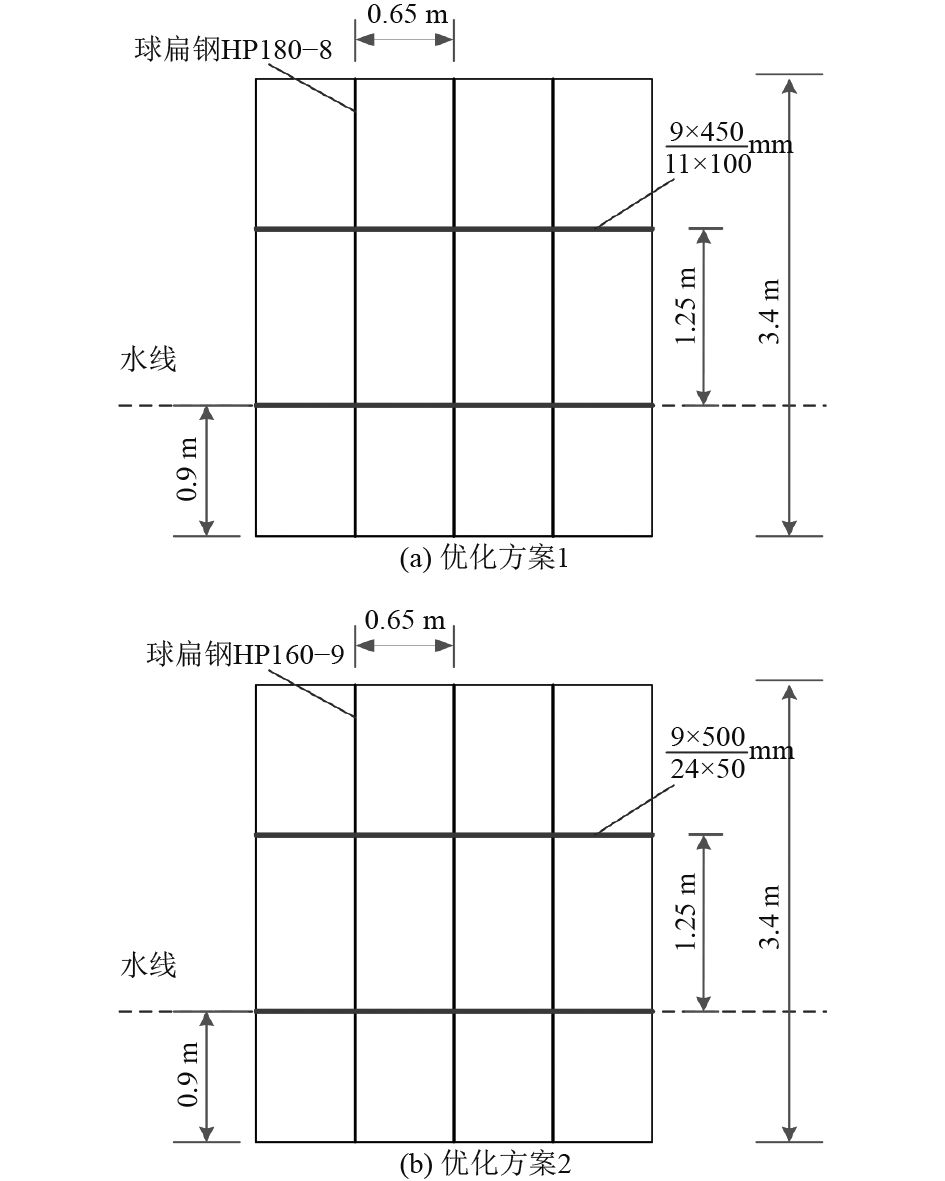图 7 优化后的板架结构图 Fig. 7 Optimization design plan of the grillages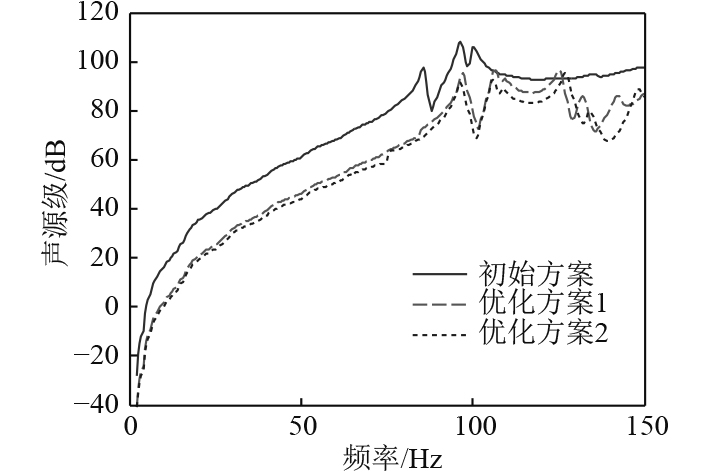图 8 内支柱体声源级对比结果 Fig. 8 Comparison results on the source level of the inner pillar表 1 计算结构方案的参数 Tab.1 Parameters of structure plan

3.2 纵向T型材为3根的优化方案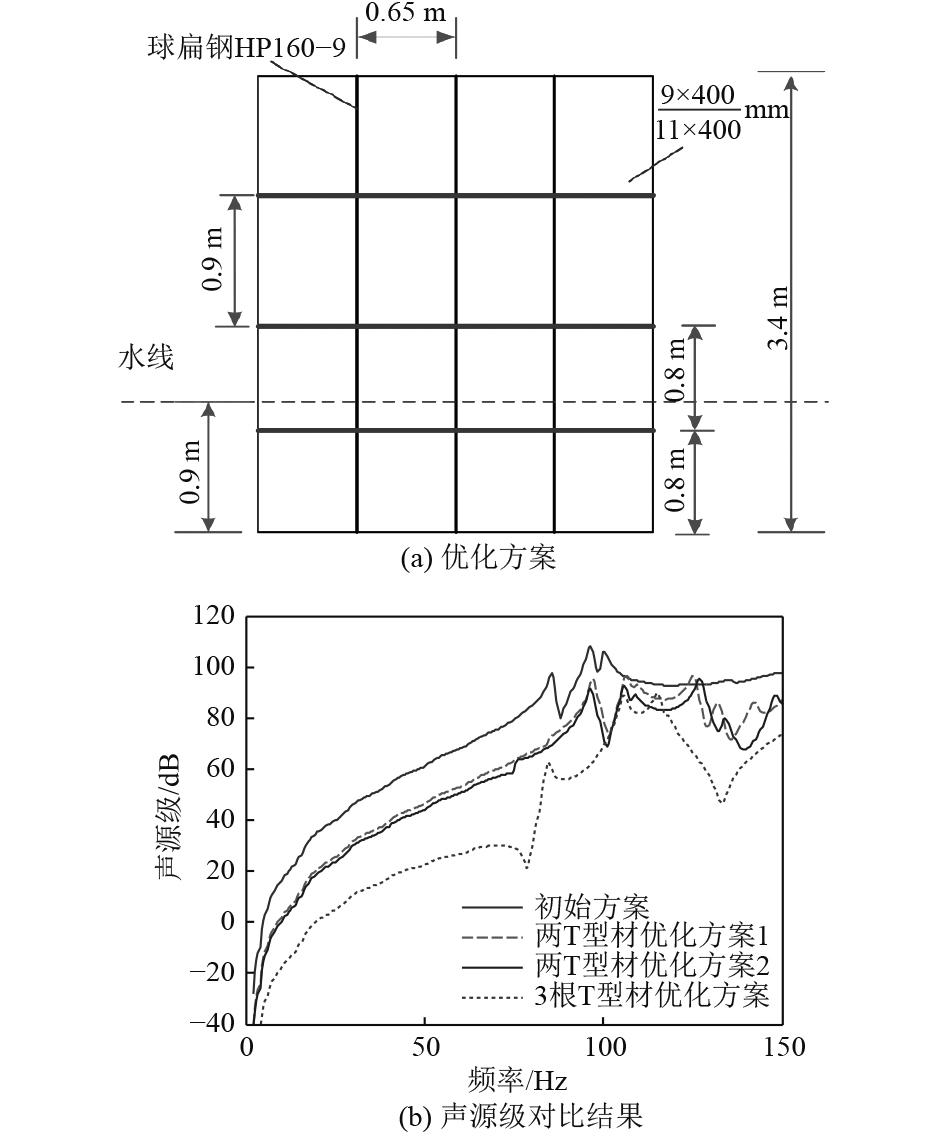图 9 内支柱体优化方案及对比结果 Fig. 9 Optimization plan and comparison results of the inner pillar

4 支柱结构低噪声阻尼材料敷设方案研究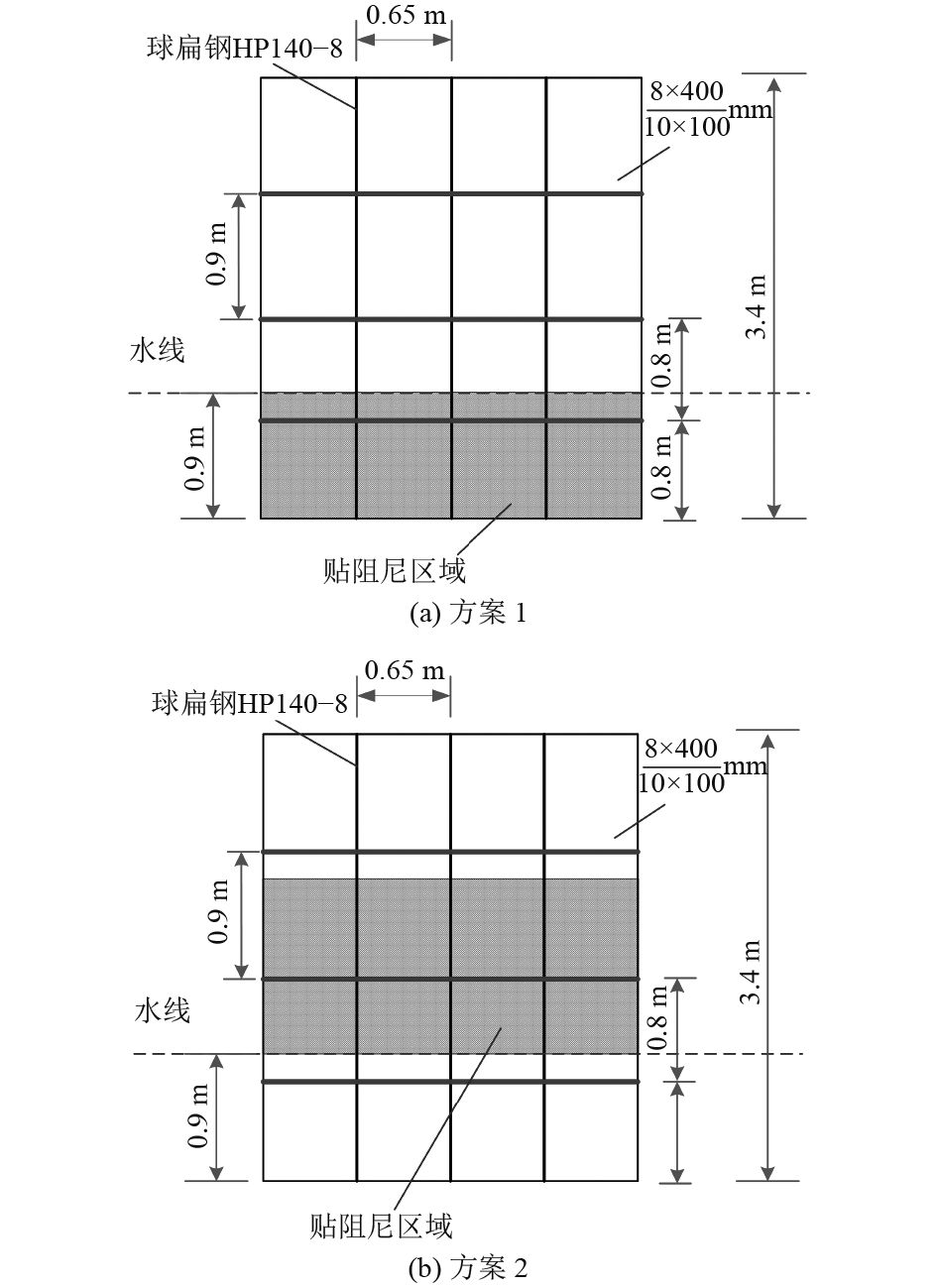图 10 阻尼材料布置图 Fig. 10 The layout of damping material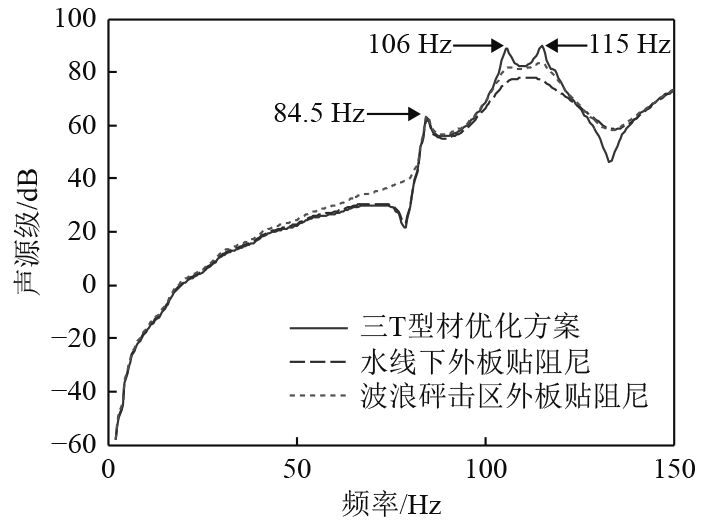图 11 声源级对比结果 Fig. 11 Comparison results of source level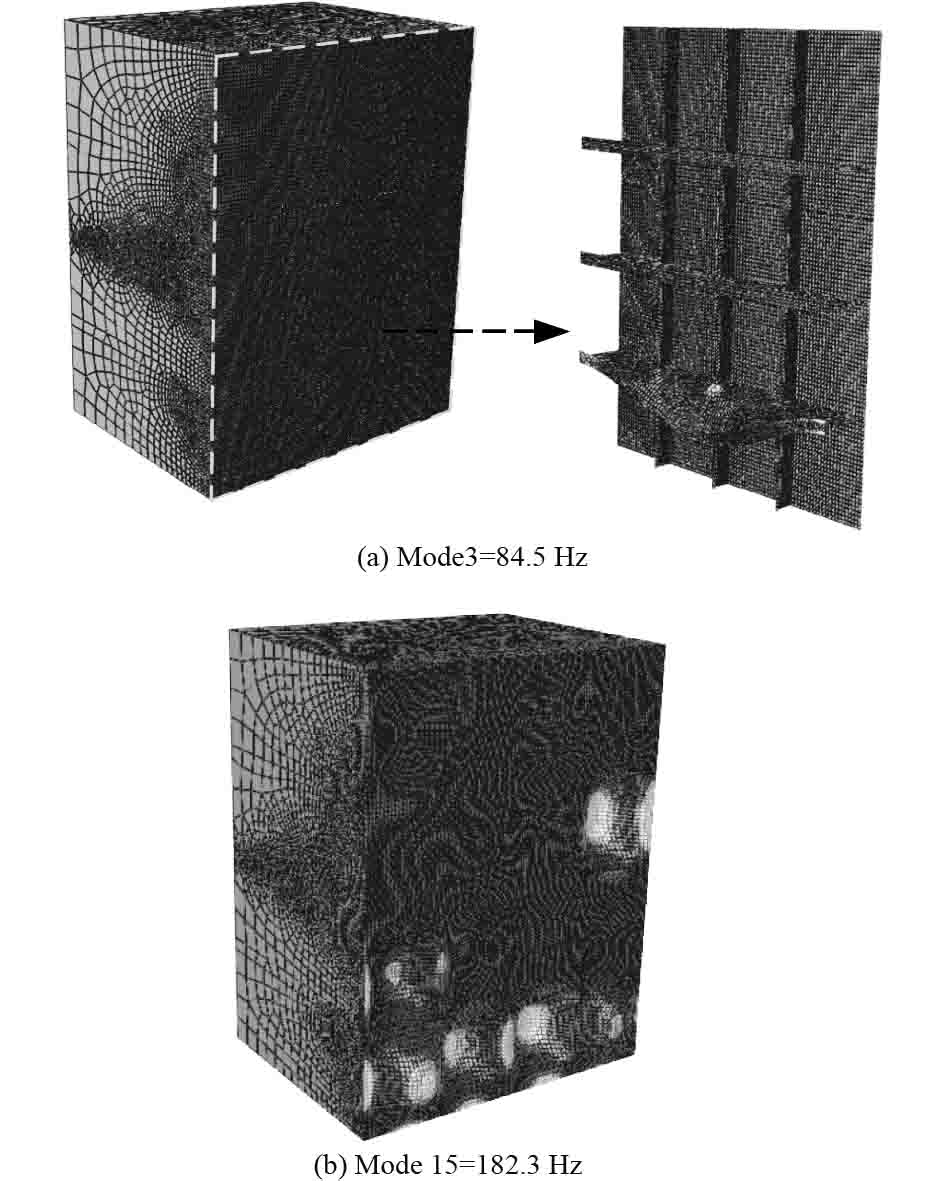图 12 对声辐射峰值贡献最大的干模态振型 Fig. 12 Dry mode shapes with the largest contribution to acoustic radiation peaks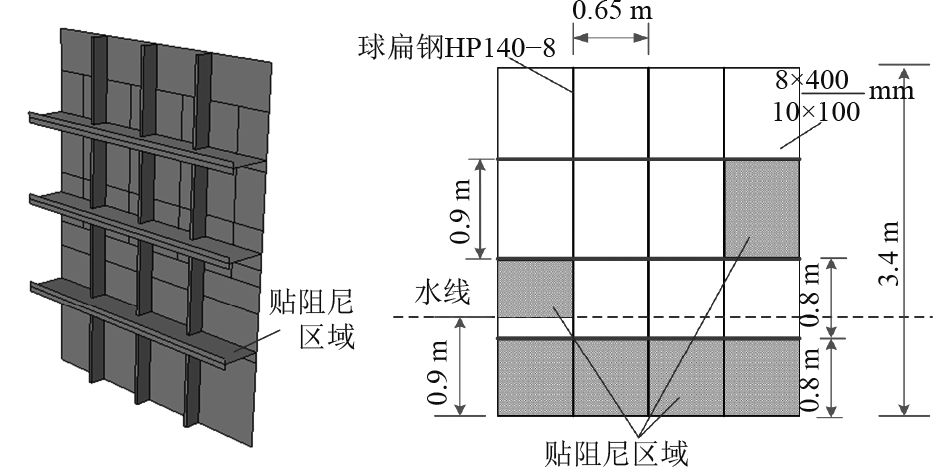图 13 阻尼敷设方案布置图 Fig. 13 The layout of damping material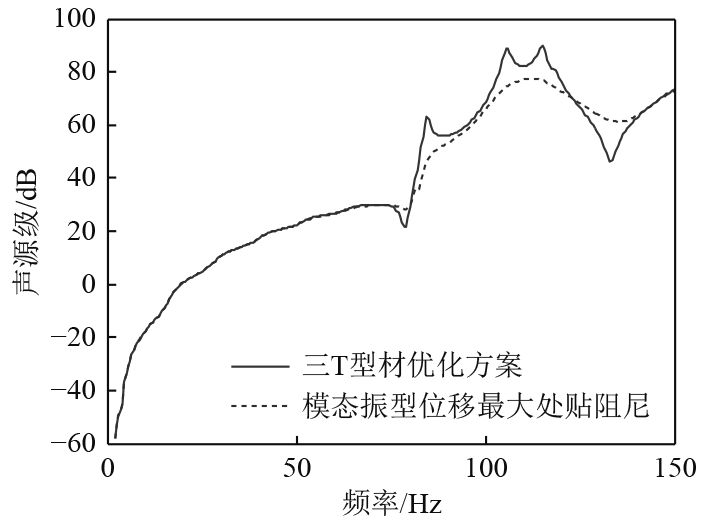图 14 声源级对比结果 Fig. 14 Comparison results of source level
5 结　语

  林吉如, 石理国, 尤国红, 等. 小水线面双体船波浪设计载荷估算方法[J]. 中国造船, 2008, 49(3): 104-111. LIN Ji-ru, SHI Li-guo, YOU Guo-hong, et al. The method for evaluating the design wave loads on SWATH ship[J]. Shipbuilding of China, 2008, 49(3): 104-111. DOI:10.3969/j.issn.1000-4882.2008.03.014  龚家烨, 李云波, 常赫斌. 小水线面双体船阻力及航态预报方法[J]. 哈尔滨工程大学学报, 2015, 36(11): 1427-1432. GONG Jia-ye, LI Yun-bo, CHANG He-bin. Prediction method for resistance and hull gesture of a small waterplane area twin hull[J]. Journal of Harbin Engineering University, 2015, 36(11): 1427-1432.  黄毅. 小水线面双体船噪声环境特性研究[D]. 哈尔滨: 哈尔滨工程大学, 2011. HUANG Yi. Research on noise environment characteristics of small waterplane area twin hull[D]. Harbin: Harbin Engineering University, 2011.  GD 06-2005, 小水线面双体船指南[S]. 中国船级社, 2005. GD 06-2005, Guidelines small waterplane area twin hull craft[S]. China Classification Society, 2005.  蔡小阳. 小水线面双体船的运用与发展[J]. 舰船科学技术, 2012, 34(增刊2): 61–64. CAI Xiao-yang. SWATH vessels application and development[J]. Ship Science and Technology, 2012, 34(S2): 61–64.  邹明松, 刘艳敏, 祁立波. 舷间充水双层弹性薄球壳结构声辐射研究[J]. 船舶力学, 2013, 17(1-2): 155-163. ZOU Ming-song, LIU Yan-min, QI Li-bo. Structural-acoustic radiation of elastic thin spheric double-shell with contained water[J]. Journal of Ship Mechanics, 2013, 17(1-2): 155-163.  SKELTON E A. JAMES J H. Theoretical acoustics of underwater structures[M], Imperial College Press, London, 1997.  何祚镛. 结构振动与声辐射[M]. 哈尔滨: 哈尔滨工程大学出版社, 2001. HE Zuo-yong. Structural vibration and acoustic radiation[M]. Harbin: Harbin Engineering University press, 2001.  WU Y S. Hydroelasticity of floating bodies[D]. Ph. D. Thesis, London, Brunel University, 1984.  PRICE W G, WU Y S. Hydroelasticity of marine structure[C]//In: Sectional Lecture S-10, the 16th In. Congress of Theoretical and Applied Mechanics (IUTAM), Lyngby, Denmark, 1984.  邹明松, 吴有生, 沈顺根, 等. 考虑航速及自由液面影响的声介质中三维结构水弹性力学研究[J]. 船舶力学, 2010, 14(11): 1304-1311. ZOU Ming-song, WU You-sheng, Shen Shun-gen, et al. Three-dimensional hydroelasticity with forward speed and free surface in acoustic medium[J]. Journal of Ship Mechanics, 2010, 14(11): 1304-1311. DOI:10.3969/j.issn.1007-7294.2010.11.015  ZOU Ming-Song, WU You-Sheng, LIU Yan-Min. The application of three-dimensional hydroelastic analysis of ship structures in Pekeris hydro-acoustic waveguide environment[J]. Acta Mechanica Sinica, 2014, 30(1): 59-66. DOI:10.1007/s10409-013-0096-0  邹明松, 吴有生, 孙建刚, 等. 基于船舶三维声弹性理论的计算技术[J]. 声学技术, 2016, 35(6): 112-115. ZOU Ming-song, WU You-sheng, SUN Jian-gang, et al. Computational techniques based on the three-dimensional sono-elasticity of ships[J]. Technical Acoustics, 2016, 35(6): 112-115.  王甜, 黄小平, 韩正君. 球扁钢等效的方法研究[J]. 船舶工程, 2013, 35(3): 106-109. WANG Tian, HUANG Xiao-ping, HAN Zheng-jun. Equivalent investigation of flat-bulb steel[J]. Ship Engineering, 2013, 35(3): 106-109.  陶尧森. 船舶耐波性[M]. 上海: 上海交通大学出版社, 1985.  段绍伟, 向湘林, 沈蒲生. 复杂阻尼结构阻尼模型研究[J]. 振动与冲击, 2011, 30(2): 73-76. DUAN Shao-wei, XIANG Xiang-lin, SHEN Pu-sheng. Damping model of a complex structure[J]. Journal of Vibration and Shock, 2011, 30(2): 73-76. DOI:10.3969/j.issn.1000-3835.2011.02.015